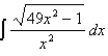# Calculus Early Transcendentals

Mathematics

## Quiz 6 : Techniques of IntegrationStudy FlashcardsLooking for Calculus Homework Help?

## Quiz 6 :Techniques of Integration

Question TypeUse the Table of Integrals to evaluate the integral to three decimal places.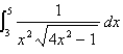Free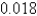Tags
Choose question tagA manufacturer of light bulbs wants to produce bulbs that last about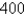hours but, of course, some bulbs burn out faster than others. Let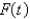be the fraction of the company's bulbs that burn out before t hours.lies between 0 and 1.Let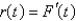. What is the value of?
Free
Multiple Choice

A

Tags
Choose question tagDetermine whether the improper integral converges or diverges, and if it converges, find its value.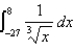Free
Multiple Choice

A

Tags
Choose question tagFind the integral.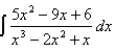Multiple Choice
Tags
Choose question tagEvaluate the integral or show that it is divergent.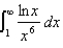Tags
Choose question tagDetermine whether the improper integral converges or diverges, and if it converges, find its value.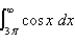Multiple Choice
Tags
Choose question tagLet a and b be real numbers. What integral must appear in place of the question mark "?" to make the following statement true?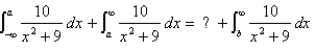Multiple Choice
Tags
Choose question tagEvaluate the integral.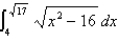Tags
Choose question tagFind the integral.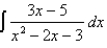Multiple Choice
Tags
Choose question tagUse Simpson's Rule to approximate the integral with answers rounded to four decimal places.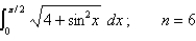Multiple Choice
Tags
Choose question tagUse Simpson's Rule to approximate the integral with answers rounded to four decimal places.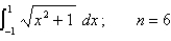Multiple Choice
Tags
Choose question tagUse the Table of Integrals to evaluate the integral.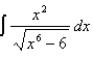Tags
Choose question tagUse the Midpoint Rule to approximate the given integral with the specified value of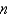. (Round your answers to four decimal places.)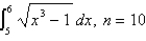Multiple Choice
Tags
Choose question tagEstimate the area of the shaded region by using the Trapezoidal Rule with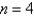. Round the answer to the nearest tenth.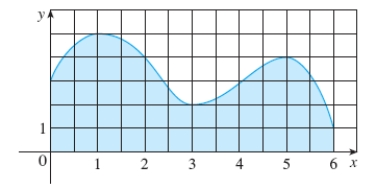Tags
Choose question tagWrite the form of the partial fraction decomposition of the rational expression. Do not find the numerical values of the constants.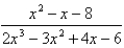Multiple Choice
Tags
Choose question tagUse the Midpoint Rule to approximate the given integral with the specified value of. (Round your answers to four decimal places.)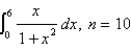Multiple Choice
Tags
Choose question tagDetermine whether the improper integral converges or diverges, and if it converges, find its value.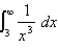Multiple Choice
Tags
Choose question tagUse a table of integrals to evaluate the integral.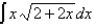Multiple Choice
Tags
Choose question tagFind the integral.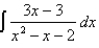Multiple ChoiceUse the Table of Integrals to evaluate the integral.